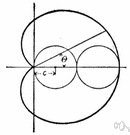# cardioid

(redirected from Cardioids)
Also found in: Thesaurus, Medical, Encyclopedia.
cardioid
The parametric equations of this cardioid are
x = -a cos θ (1 - cos θ),
y = a sin θ (1 - cos θ).

## car·di·oid

(kär′dē-oid′)
n.
A heart-shaped plane curve, the locus of a fixed point on a circle that rolls on the circumference of another circle with the same radius.

## cardioid

(ˈkɑːdɪˌɔɪd)
n
(Mathematics) a heart-shaped curve generated by a fixed point on a circle as it rolls around another fixed circle of equal radius, a. Equation: r = a(1 – cos φ), where r is the radius vector and φ is the polar angle

## car•di•oid

(ˈkɑr diˌɔɪd)

n.
a heart-shaped curve traced by a point on a circle that rolls without slipping on another equal circle. Equation: r = a (1−cosA).
[1745–55; < Greek kardioeidḗs heart-shaped]
ThesaurusAntonymsRelated WordsSynonymsLegend:
 Noun 1cardioid - an epicycloid in which the rolling circle equals the fixed circleepicycloid - a line generated by a point on a circle rolling around another circle
Translations

## cardioid

[ˈkɑːdɪˌɔɪd] n (Math) → cardioide f
Mentioned in ?
References in periodicals archive ?
The Mandelbrot set, graphically, can be divided into an infinite set of cardioids, with the largest of them located at the center of the complex plane.
Resp: 20 Hz - 20Khz, Low Cut Filter: Selectable At Rate Of 160 Hz, 3Db/Oct, Polar Pattern: Super Cardioids, Sensitivity: 38Db At 1Khz 0Db Equal To 1 V/Pa , Impedance: 120 At 1 Khz , Max.
The high-sensitivity, noise-cancelling cardioids microphone helps ensure effective team play.

Site: Follow: Share:
Open / Close